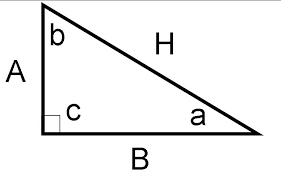CosineFor this equation, you need to find the Cosine(cos) button on your calculator. This will help you find the adjacent side of your triangle. For this image,lets say you need to find side A and you know angle b. You should set up your equation as cos(b)=A/H. Then you put cos(b)over 1. Next, cross multiply cos(b) by the length of the Hyp, or H . This should bring you to the equation A=cos(b)xH and that is how you find the Adjacent.To see how to solve for Hypotenuse see Sine Tutorial.